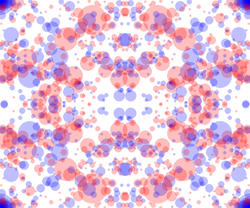I have been thinking about ways to connect number theory and computational logic. For example, Euclid's greatest common divisor algorithm can be expressed in terms of natural numbers instead of integers, which makes it easier to specify and verify in an interactive theorem prover. The arithmetic Haskell package contains a library of computational number theory algorithms that similarly operate on natural numbers and are ripe for formalization.

The image on the right is a plot of all numbers modulo the product of two primes that have small squares. Do you enjoy solving mathematical puzzles?